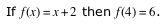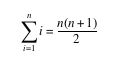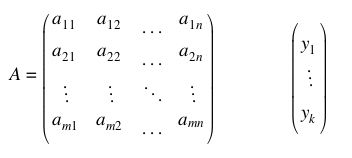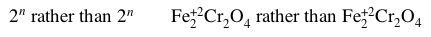## JSMath Integration

See also:
External links:
• INFOPedia - a Wikka-powered wiki with JSMath support.

JSMath is an easy-to-use script that allows one to display complex mathematics in web pages using JavaScript. The way the script is structured should make it simple to integrate into Wikka. Integration into Wikka would allow users to display complex mathematics using LaTeX markup, making Wikka useful for mathematical resources.

JSMath has a homepage here. A more thorough explanation of the way in which JSMath works is here.

Here's a quick hack to integrate JSMath in Wikka

### The code

Version 0.1

##### 1. Download and save the package
Get the package, unzip it and upload the files in the following folder in your Wikka directory:

3rdparty/plugins/jsmath/

##### 2. Modify wikka.config.php
Add the JSMath options to the configuration file:

original
1.     "geshi_path" => "3rdparty/plugins/geshi",
2.     "geshi_languages_path" => "3rdparty/plugins/geshi/geshi",

modified
1.     "geshi_path" => "3rdparty/plugins/geshi",
2.     "geshi_languages_path" => "3rdparty/plugins/geshi/geshi",
3.     "enable_jsmath" => "1",
4.     "jsmath_path" => "3rdparty/plugins/jsmath",

##### 3. Modify actions/header.php

Insert the JSMath call in actions/header.php:

original
1. <body <?php echo $message ? "onLoad=\"alert('".$message."');\" " : "" ?> >
2. <div class="header">

modified
1. <body <?php echo $message ? "onLoad=\"alert('".$message."');\" " : "" ?> >
2. <?php
3.     if (($this->GetMethod() == 'show') && ($this->GetConfigValue('enable_jsmath') == 1) && file_exists($this->config['jsmath_path'].'/jsMath.js')) { 4. echo '<script src="'.$this->config['jsmath_path'].'/jsMath.js" type="text/javascript"></script>';
5.     }
6. ?>
7. <div class="header">

##### 4. Modify actions/footer.php

Insert the call to the JSMath formatter function at the end of actions/footer.php:

<?php
if (($this->GetMethod() == 'show') && ($this->GetConfigValue('enable_jsmath') == 1) && file_exists($this->config['jsmath_path'].'/jsMath.js')) { echo '<script type="text/javascript">jsMath.Process()</script>'; } ?> ##### 5. Test it To add math in a Wikka page just use the math class with embedded HTML: For inline math, use: <span class="math">Here goes LaTeX code</span> For math blocks, use: <div class="math">Here goes LaTeX code</div> Try for example: ""If <span class="math"> f(x) = x+2 </span> then <span class="math"> f(4) = 6 </span>. "" ""<div class="math"> \sum_{i=1}^{n} i = {n(n+1)\over 2} </div> "" ""<div class="math"> A = \pmatrix{a_{11} & a_{12} & \ldots & a_{1n}\cr a_{21} & a_{22} & \ldots & a_{2n}\cr \vdots & \vdots & \ddots & \vdots\cr a_{m1} & a_{m2} & \ldots & a_{mn}} \qquad\qquad \pmatrix{y_1\cr\vdots\cr y_k}</div>"" ""<span class="math"> 2^{\raise1pt n}\hbox{ rather than }2^n \qquad {\rm Fe_2^{+2} Cr_2^{\vphantom{+2}}O_4^{\vphantom{+2}}} \hbox{ rather than } {\rm Fe_2^{+2} Cr_2 O_4} </span>"" if the JSMath engine works correctly the code above should be rendered as:(the above examples are pictures taken from a local installation; JSMath outputs math as formatted text) ##### Latex Style Wiki Tags A short hack at formatters/wakka.php will lead to some much nicer Latex Style tags for entering & exiting math mode. Edit formatters/wakka.php and add the following lines at the relevent line numbers (line numbers from original wakka.php. so work from the bottom up) 1. static$trigger_math_inline = 0;
2.                static $trigger_math_block = 0; 1. if ($trigger_math_inline % 2) echo('</span>');
2.                        if ($trigger_math_block % 2) echo('</div>'); 1. // Math Inline 2. else if ($thing == "$$") 3. { 4. return(++trigger_math_inline % 2 ? "<span class=\"math\">" : "</span>"); 5. } 6. else if (thing == "$" && trigger_math_block == 0) 7. { 8. trigger_math_block++; 9. return "<div class=\"math\">\n"; 10. } 11. else if (thing == "$" && trigger_math_block == 1) 12. { 13. trigger_math_block = 0; 14. return "\n</div>\n"; 15. }  "\\\\|". # Math inline "\\\\\$|\\\\\$|". # Math Block then use$$ 1 +1  for inline markup and $1+1$ for block markup.

### To do

• create a dedicated formatter;
• handle font-related error messages;
• add support for TeX fonts.

CategoryDevelopment3rdParty CategoryDevelopmentFormatters
There are 9 comments on this page. [Show comments]